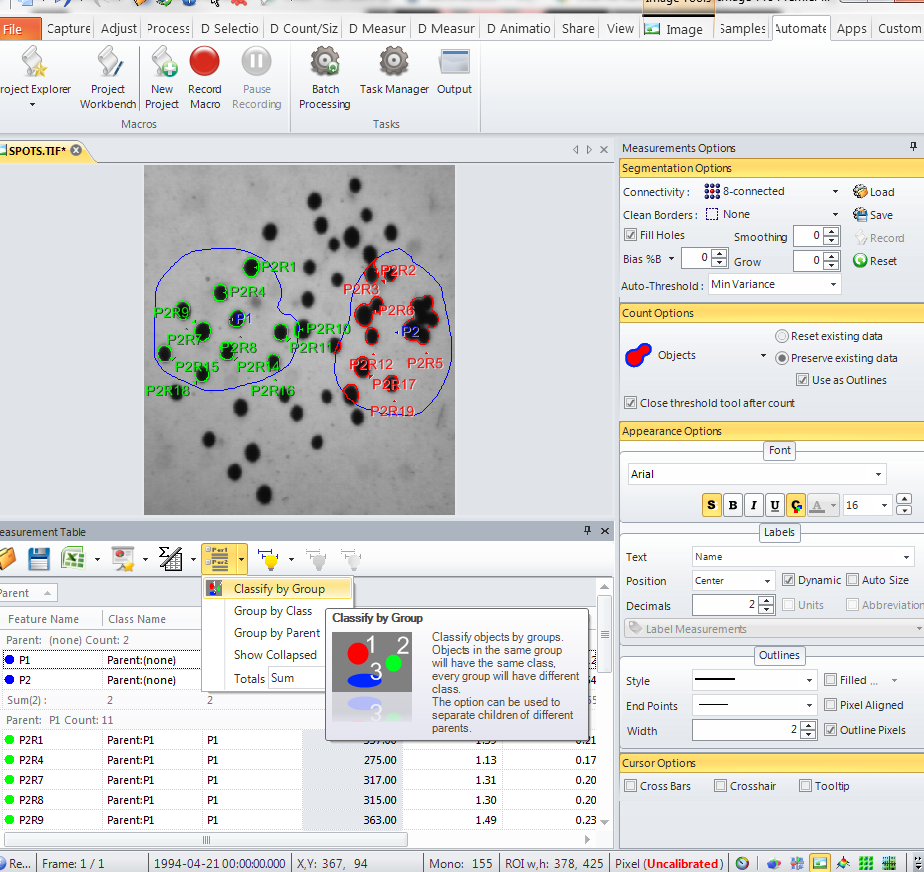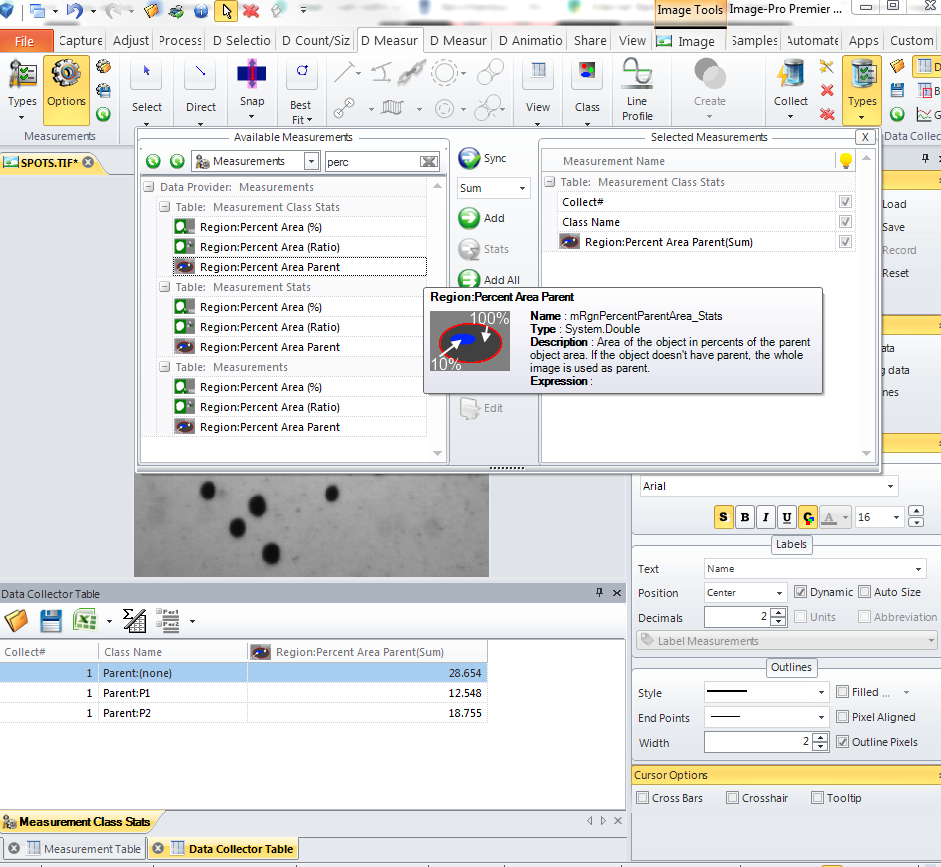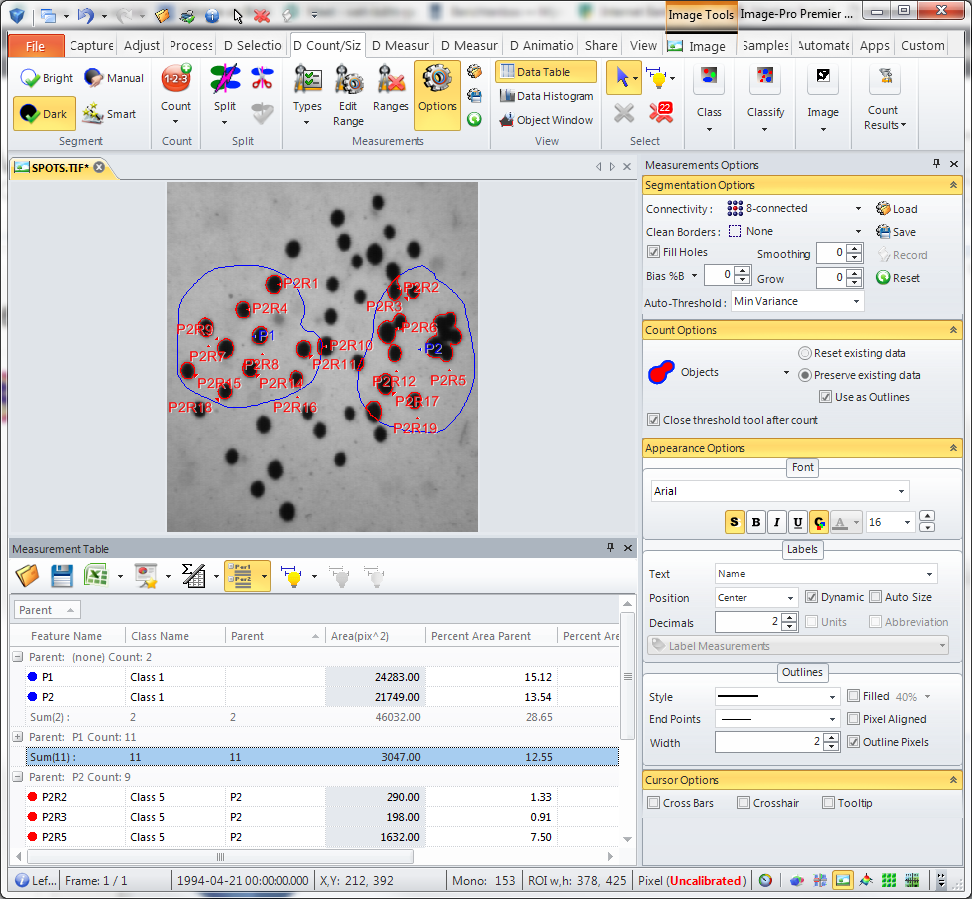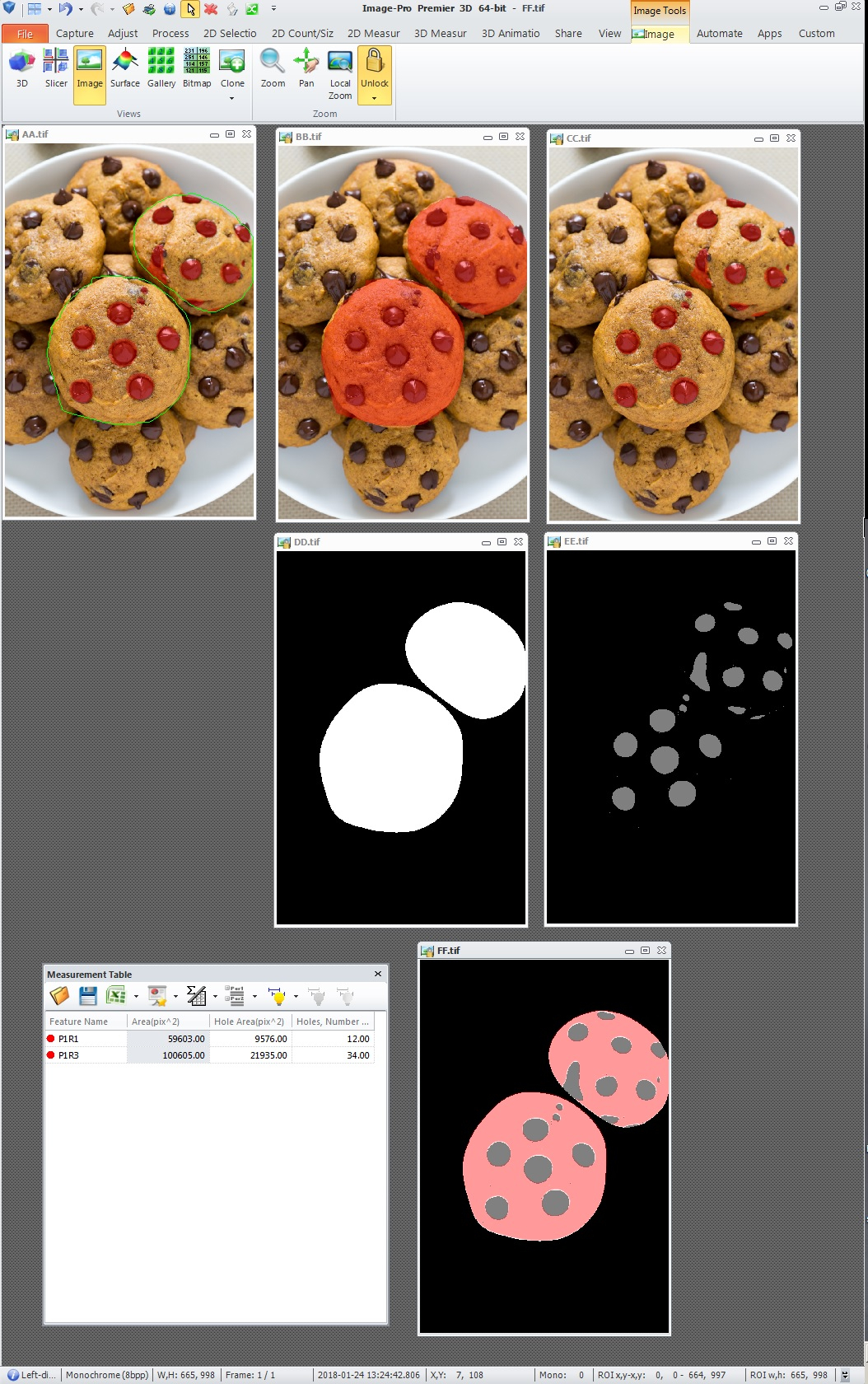Retrieve Parent-Child data

Hi Folks,

I use the first measurement as ROIs and then have a second from which I just have to retrieve the RgnPerArea...

So I have two classes, the one with the parents (from which I need length, width....) and the second with the children.

I can retrieve the values to the parents well by this code...
Public Function GetClassDataT() As SimpleScript
GetClassDataT = New SimpleScript

Dim im As McImage=ThisApplication.ActiveImage
If im Is Nothing Then Exit Function 'no image
Dim md As McMMData=im.MeasurementsData
'If md.Count=0 Then Exit Function'no data
Dim nClasses As Integer=classes.Items.Count

With Automate.ScriptingCommands.CodeCommand(GetClassDataT)
If .Run() Then

For Each cl As MMClassDescr In classes.Items.Values

For Each sf As McMMSubFeature In md.SubFeatures

If sf.Value(eMeasures.AnyClass) = 1 Then

If sf.Value(eMeasures.AnyClass)=cl.Value Then TWidth = sf.value(eMeasures.RgnWidth)
If sf.Value(eMeasures.AnyClass)=cl.Value Then TLength= sf.value(eMeasures.RgnLength)
If sf.Value(eMeasures.AnyClass)=cl.Value Then SumTSize = SumTSize + sf.value(eMeasures.RgnArea)

end if end with

• dhavas,

You could use Parent and PercentAreaParent measures in a macro to get the sum, like this

Public Sub ParentMeasures
Dim dict As New System.Collections.Generic.Dictionary(Of String,Double)
For Each sf As McMMSubFeature In ThisApplication.ActiveImage.MeasurementsData.Subfeatures
Dim sParent As String=sf.Value(eMeasures.AnyParentName)
Dim v As Double=sf.Value(eMeasures.RgnPercentParentArea)
If Not dict.ContainsKey(sParent) Then
Else
dict(sParent)=dict(sParent)+v'calculate sum
End If
Next
For Each kv As System.Collections.Generic.KeyValuePair(Of String,Double) In dict
Debug.Print (String.Format("Parent {0} - Percent Area Parent Sum = {1}",kv.Key,kv.Value))
Next
End Sub

The easier way is to Classify by Group (after grouping by Parent) , features of different parents will be assigned to different classesand then collect data to Data Collector, Measurements Class Stats table, Percent Area Parent Sum will give you the value you needYuri

• Sorry was not complete...
again

How can I get the values of RngPerArea o the children now for each of the parents

Public Function GetClassDataT() As SimpleScript
GetClassDataT = New SimpleScript

Dim im As McImage=ThisApplication.ActiveImage
If im Is Nothing Then Exit Function 'no image
Dim md As McMMData=im.MeasurementsData
'If md.Count=0 Then Exit Function'no data
Dim nClasses As Integer=classes.Items.Count

dim TWidth, TLength, SumTSize

With Automate.ScriptingCommands.CodeCommand(GetClassDataT)
If .Run() Then

For Each cl As MMClassDescr In classes.Items.Values

For Each sf As McMMSubFeature In md.SubFeatures

'each parent in class 1
If sf.Value(eMeasures.AnyClass) = 1 Then

If sf.Value(eMeasures.AnyClass)=cl.Value Then TWidth = sf.value(eMeasures.RgnWidth)
If sf.Value(eMeasures.AnyClass)=cl.Value Then TLength= sf.value(eMeasures.RgnLength)
If sf.Value(eMeasures.AnyClass)=cl.Value Then SumTSize = SumTSize + sf.value(eMeasures.RgnArea)

'XXXXXXXXXXX    HERE I'D NEED THE percent area or area of the children for each parent.
' in the end I need the % area of the Size of the parent covered by labeling within the parent's outlines

end if
next
next

end if
end with

'MANY THANKS
• dhavas,

There is an easier way to measure Percent Area Parent, which is mentioned in some previous topics:
http://forums.mediacy.com/discussion/comment/1113#Comment_1113
http://forums.mediacy.com/discussion/comment/1155#Comment_1155

In short: you just create your parent areas as Measurements (not ROIs), manually or using automatic segmentation, and then Activate "Preserve existing data", "Use as Outlines" and Count objects inside. Then check "Percent Area Parent" statistics, you can optionally group the table "By Parent". Here is the screenshot:Yuri
• Hi Yuri,

This is exactly what I do...

I need the code to retrieve the data, since I need to calculate with them.

So how do I get the statistics of the RngPercentParentArea (I found this one :-)) for each parent separately.

(I'm measuring tumors and  cellular content within).

My problem is that when I retrieve the single values, they are not sorted to parent. So if I get the class data of the second class (the children) then they are not easy to allocate to the parent so that I can sum them up.

I'm sure there is a relative simple way, but I don't come on it...

Cheers

Dan

• 2018-01-24-131834

Dhavas --

There may be a more sophisticated way to do this but I would use MASKS and HOLE AREA and HOLES NUMBER.

A path through this is shown below where:

AA is the ORIGINAL IMAGE with the ROIs and the AREAS found within the ROIs
BB is the ORIGINAL IMAGE with the ROIs converted to MEASUREMENTS using the FEATURE MANAGER
CC is the ORIGINAL IMAGE with the AREAS and no ROIs
DD is the MASK of BB
EE is the MASK of CC (plus a division by 2 to change 255 to 128)
FF is DD - EE plus a MONO THRESHOLD of 130 to 255 and a COUNT.

I think the information you want is now available in the DATA TABLE.

The only issue I see is if one of your AREAS is at the boundary of the ROI.  I think you can deal with this though by maybe DILATING the DD MASK by a pixel or two or by eroding the EE MASK by a pixel or two.

I hope this information is helpful.

-- Matt• Hi Matt,

I just saw this answer - working  a lot with masks and this would definitely work.

But there was in the end some code way to retrieve.

Here a short version of it:

Public Function GetClassDataT() As SimpleScript
GetClassDataT = New SimpleScript

Dim im As McImage=ThisApplication.ActiveImage
If im Is Nothing Then Exit Function 'no image
Dim md As McMMData=im.MeasurementsData
'If md.Count=0 Then Exit Function'no data
Dim nClasses As Integer=classes.Items.Count

Dim n, count, area, ClDescription
ReDim count(nClasses) As Double
ReDim area(nClasses) As Double

Dim nObj As Integer = 0
Dim AreaSum As Double = 0
Dim currparent, tempparent

Dim dict As New System.Collections.Generic.Dictionary(Of String,Double)
With Automate.ScriptingCommands.CodeCommand(GetClassDataT)
If .Run() Then

For Each sf As McMMSubFeature In md.SubFeatures Dim sParent As String=sf.Value(eMeasures.AnyParentName) Dim v As Double=sf.Value(eMeasures.RgnPercentParentArea) If Not dict.ContainsKey(sParent) Then dict.Add(sParent,v) Else dict(sParent)=dict(sParent)+v'calculate sum End If Next For Each kv As System.Collections.Generic.KeyValuePair(Of String,Double) In dict 'Debug.Print (String.Format("Parent {0} - Percent Area Parent Sum = {1}",kv.Key,kv.Value)) currparent = kv.Key TCell = kv.Value 'go for subfeatures of Parents For Each sfP As McMMSubFeature In md.SubFeatures ' tempparent = sfP.Name If Trim(tempparent) = Trim(currparent) Then 'DO SOMETHING End If 'this very parent or tumor Next 'sfP Next 'kv Tcell determination and also tumor
End If
End With

End Function
• 2018-05-24-104735

Dhavas --

I am glad that you were able to develop a method and code it to provide your solution.

Thank you for posting the code here so others can follow your path and alter it as suits their needs.

-- Matt